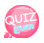# Global and local variables.

Today, let's learn about global variables and local variables.
First, let's look at functions before describing global variables and local variables.
You know f (x), well... you don't have to know it.
I declare a function to do a certain action and enter a specific action in the function.

```function function_name(){
}```

The above source is the function declaration.
First, write down the funciton for the function declaration and the name of the function.
Then open and close the parentheses, then open and close the brackets and write the statements to be executed inside the square brackets.
For example, let's call the function ipad.

```function ipad(){
}```

As abobe, after function, write ipad, open and close parentheses, then open and close brackets. Then a function called ipad is created.
will it open after creatng a funtion? No. You must call the function separately. When you call the function, waht you should use is the function name();

### Funtion Call

```Funtion name();
For exmaple, if you created the funtion ipad as above,

Let's test it .

First let's create a function. Function we will use here is to outputs hello world.

```function hello(){
document.write("Hello world");
}```

This is a function, but no code to call it. Test it and look at the result. The result is normal if there is nothing.

```<!DOCTYPE html>
<html>
<meta charset="utf-8" />
<title>JavaScript</title>
<script>
function hello(){
document.write("Hello world");
}
</script>
<body>
</body>
</html>```

If you try typing the above source or you want to test it by clicking the View Source on the web button, you will see that nothing appears on the result screen.
Let's check the result using the following function call statement.

`    hello();`

The above source is a function that calls a function called hello. Then a function called hello is called, so the output statement inside the hello function executes.

Let's see!

```<!DOCTYPE html>
<html>
<meta charset="utf-8" />
<title>JavaScript</title>
<script>
function hello(){
document.write("Hello world");
}
hello();
</script>
<body>
</body>
</html>```

If you look at the above source, you can see that the hello function works and outputs the word hello world. Now that we have time to look at global variables and local variables, we will end the explanation of the functions here. ^ - ^

### var

Var is used to declare a variable.

```When declaring a variable
Var variable name = value of the variable;```

It can be used without attaching var.
As you can see from the name, global variables and local variables are available everywhere, and local variables are variables that are used only in specific regions.

The specific area referred to here is a function. A function that only works inside a specific function that you have created is a local function that you can not use outside of the function. However, global functions can be used in places other than these functions. To make it easier to understand, let's create one using css.

<!DOCTYPE html>
<html>
<title>JavaScript</title>
<script>
a = 10;

function hello(){
hello = "Hello World";

document.write(hello);
}

</script>
<body>
</body>
</html>

The red area is the space in which the global variable is recognized. So the name is also a global variable. ^ - ^

The blue area is the space where local variables are recognized. It works only within functions. So it is a local (local) variable. ^ - ^

enough. Let's test it by declaring a direct variable outside function and in function. The following example declares the global variable glo_var, declares a local variable local_var in the function, and outputs the glo_var variable using the output statement outside the function.

```<!DOCTYPE html>
<html>
<meta charset="utf-8" />
<title>JavaScrpt</title>
<style type="text/css">
</style>
<script type="text/javascript">

glo_var = 10;
function hello(){
local_var = 20;
}

document.write(glo_var);
</script>
<body>
</body>
</html>```

If you run the above source, you can see that the value of the variable glo_var is 10. So, in the source above, change the output statement variable to a local variable and see if the value should be output. The result ,of course, will not be printed since it is local variable, but let's see and understand it.

```<!DOCTYPE html>
<html>
<meta charset="utf-8" />
<title>JavaScript</title>
<style type="text/css">
</style>
<script type="text/javascript">

glo_var = 10;
function hello(){
local_var = 20;
}
document.write(local_var);

</script>
<body>
</body>
</html>```

If you run the above source, you will see that nothing is printed in the result. The reason is that we run the local variable outside the function. ^^

### The difference between prefixing a variable name with a var or not

It is a global variable that is prefixed with a var before the global variable outside the function.
However, it is different in functions.

#### If there is a var before the local variable, the local variable only works inside the function. If there is no var before the local variable, it becomes a global variable after the function is called once.

Let's look at the following example.
The following example is a function that prints the variable local_var2, which is not with a var variable,and could be pricted outside the function.
A variable that does not have a var will become a global variable once it is called. The following source does not have a call to the hello function, so the result will not be output. ^^

```<!DOCTYPE html>
<html>
<meta charset="utf-8" />
<title>JavaScript</title>
<style type="text/css">
</style>
<script type="text/javascript" src="https://code.jquery.com/jquery-3.2.0.min.js" ></script>
<script type="text/javascript">

glo_var = 10;
function hello(){
var local_var = 20;
local_var2 = 30;
}
document.write(local_var2);

</script>
<body>
</body>
</html>```

The source below is the declaration of hello() function call from the source above. By calling the function, local_var2 variable becomes a global variable, so it shows the result.

```<!DOCTYPE html>
<html>
<meta charset="utf-8" />
<title>JavaScript</title>
<style type="text/css">
</style>
<script type="text/javascript" src="https://code.jquery.com/jquery-3.2.0.min.js" ></script>
<script type="text/javascript">

glo_var = 10;
function hello(){
var local_var = 20;
local_var2 = 30;
}
hello();
document.write(local_var2);

</script>